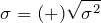# Standard Deviation

March 29, 2018

The standard deviation of a set of numbers, xnn = 1, …, N, is given in Equation 6:

(1)Equation 6

The standard deviation is a measure of the variability of a signal about its mean value. For a vibration signal with a mean value of zero, the standard deviation is equal to the RMS (root-mean-square) value of the signal. Referring back to the vibration signal used in Figures 3.1 and 3.2, the standard deviation and RMS level have a value of 0.073 G.

The variance and standard deviation play an important role in determining the confidence interval—or, conversely, the uncertainty—of a statistical measure. This is discussed further in the lesson on Confidence Intervals.